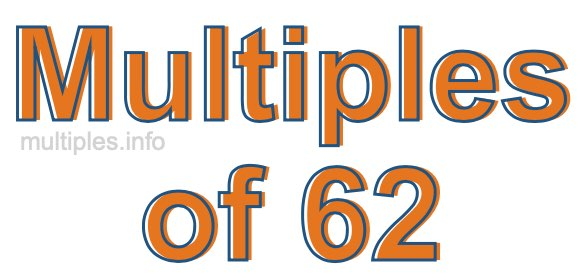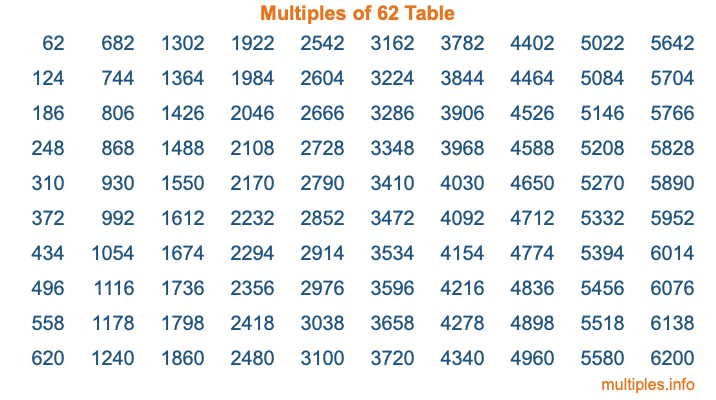Multiples of 62Welcome to the Multiples of 62 page. Here we will first teach you everything you will ever need to know about the multiples of 62, and then give you a study guide summary of everything we taught you to make sure you remember it all. Use this page to look up facts and learn information about the multiples of 62. This page will make you a multiples of sixty-two expert!

Definition of Multiples of 62
Multiples of 62 are all the numbers that when divided by 62 equal an integer. Each of the multiples of 62 are called a multiple. A multiple of 62 is created by multiplying 62 by an integer.

Therefore, to create a list of multiples of 62, you start with 1 multiplied by 62, then 2 multiplied by 62, then 3 multiplied by 62, and so on for as long as you want. Thus, the list of the first five multiples of 62 is 62, 124, 186, 248, and 310. To see a larger list of multiples of 62, see the printable image of Multiples of 62 further down on this page. We also have a category where you can choose any nth multiple of 62.

Multiples of 62 Checker
The Multiples of 62 Checker below checks to see if any number of your choice is a multiple of 62. In other words, it checks to see if there is any number (integer) that when multiplied by 62 will equal your number. To do that, we divide your number by 62. If the the quotient is an integer, then your number is a multiple of 62.

Is  a multiple of 62?

Least Common Multiple of 62 and ...
A Least Common Multiple (LCM) is the lowest multiple that two or more numbers have in common. This is also called the smallest common multiple or lowest common multiple and is useful to know when you are adding our subtracting fractions. Enter one or more numbers below (62 is already entered) to find the LCM.

Check out our LCM Calculator if you need more details about the Least Common Multiple or if you need the LCM for different numbers for adding and subtraction fractions.

nth Multiple of 62
As we stated above, 62 is the first multiple of 62, 124 is the second multiple of 62, 186 is the third multiple of 62, and so on. Enter a number below to find the nth multiple of 62.

th multiple of 62

Multiples of 62 vs Factors of 62
62 is a multiple of 62 and a factor of 62, but that is where the similarities end. All postive multiples of 62 are 62 or greater than 62. All positive factors of 62 are 62 or less than 62.

Below is the beginning list of multiples of 62 and the factors of 62 so you can compare:

Multiples of 62: 62, 124, 186, 248, 310, etc.

Factors of 62: 1, 2, 31, 62

As you can see, the multiples of 62 are all the numbers that you can divide by 62 to get a whole number. The factors of 62, on the other hand, are all the whole numbers that you can multiply by another whole number to get 62.

It's also interesting to note that if a number (x) is a factor of 62, then 62 will also be a multiple of that number (x).

Multiples of 62 vs Divisors of 62
The divisors of 62 are all the integers that 62 can be divided by evenly. Below is a list of the divisors of 62.

Divisors of 62: 1, 2, 31, 62

The interesting thing to note here is that if you take any multiple of 62 and divide it by a divisor of 62, you will see that the quotient is an integer.

Multiples of 62 Table
Below is an image of the first 100 multiples of 62 in a table. The table is in chronological order, column by column. The first column has the first ten multiples of 62, the second column has the next ten multiples of 62, and so on.The Multiples of 62 Table is also referred to as the 62 Times Table or Times Table of 62. You are welcome to print out our table for your studies.

Negative Multiples of 62
Although not often discussed or needed in math, it is worth mentioning that you can make a list of negative multiples of 62 by multiplying 62 by -1, then by -2, then by -3, and so on, to get the following list of negative multiples of 62:

-62, -124, -186, -248, -310, etc.

Multiples of 62 Summary
Below is a summary of important Multiples of 62 facts that we have discussed on this page. To retain the knowledge on this page, we recommend that you read through the summary and explain to yourself or a study partner why they hold true.

There are an infinite number of multiples of 62.

A multiple of 62 divided by 62 will equal a whole number.

62 divided by a factor of 62 equals a divisor of 62.

The nth multiple of 62 is n times 62.

The largest factor of 62 is equal to the first positive multiple of 62.

62 is a multiple of every factor of 62.

62 is a multiple of 62.

A multiple of 62 divided by a divisor of 62 equals an integer.

62 divided by a divisor of 62 equals a factor of 62.

Any integer times 62 will equal a multiple of 62.

Multiples of a Number
Here you can get the multiples of another number, all with the same attention to detail as we did for multiples of 62 on this page.

Multiples of
Multiples of 63
Did you find our page about multiples of sixty-two educational? Do you want more knowledge? Check out the multiples of the next number on our list!